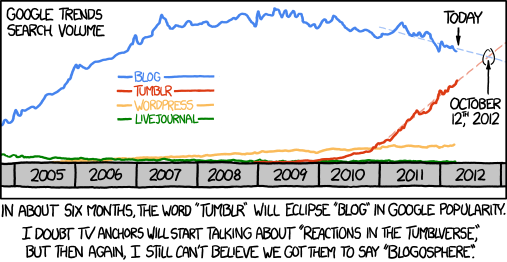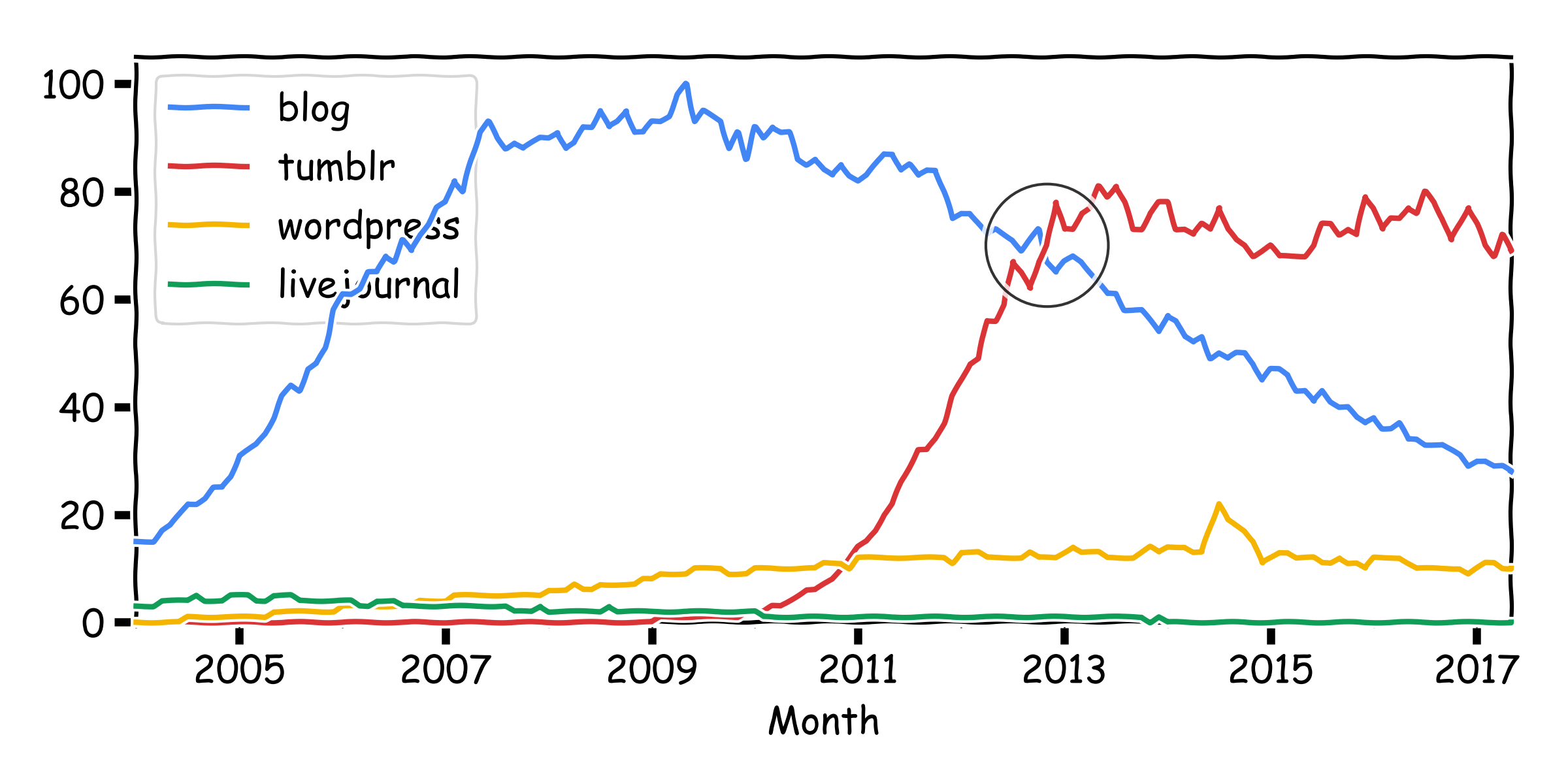# XKCD + Google Trends, 5 Years Later

Posted on

Back in 2012 XKCD #1043 presented data from Google Trends, predicting that searches for “tumblr” would overtake “blog” on 12th October 2012:Fast-forward to present (2017) and we can see the prediction was a good, with “tumblr” overtaking “blog” sometime between October and November:

The data is available in CSV format and can be displayed easily using Pandas and Matplotlib. The XKCD extension for Matplotlib even gives it that XKCD-feel.Interestingly, the “blog” trend has continued but “tumblr” has stabilized (for now).

``````import pandas
import matplotlib.pyplot as plt

plt.xkcd()

# prepare the data
df.index = df["Month"].apply(lambda x: pandas.Period(x, freq="M"))
del(df["Month"])
df.columns = [x.split(":") for x in df.columns]

# draw the plot
colors = ["#4285F4","#DB3437","#F4B400","#0F9D58"]
df.plot(figsize=(8, 4), color=colors, clip_on=False, zorder=100)
plt.plot(
pandas.Period("2012-11"), 70,
"o", zorder=200, markersize=45, alpha=0.8, markeredgecolor="k", color='none')
plt.ylim(0, None)
plt.legend(loc="upper left")
plt.tight_layout()